GROUP THEORY TUTORIAL

# What's new in GTPack 1.3

GTPack version 1.3 was released December, 2020.

Angular Momentum Package

GTPack 1.3 comes with a new package for angular momentum operators and representations.

 GTJx gives the x component of the total angular momentum operator GTJy gives the y component of the total angular momentum operator GTJz gives the z component of the total angular momentum operator

The components of the total angular momentum operator in terms of matrix representations acting on a finite sub space indexed by the total angular momentum quantum number J.

Components of the total angular momentum operator
 In:=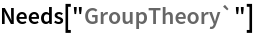In:=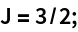In:=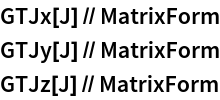Out//MatrixForm=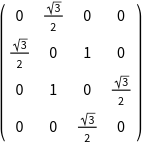Out//MatrixForm=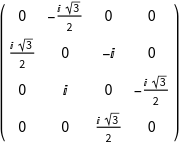Out//MatrixForm=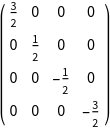Verify commutation relations.
 In:=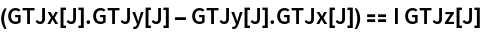Out=In:=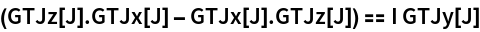Out=In:=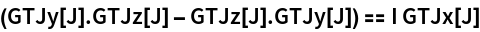Out=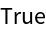GTJplus gives the raising operator GTJminus gives the lowering operator

The raising operator acts as J+|j; m> =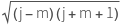|j; m+1>

The lowering operator acts as J-|j; m> =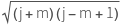|j; m-1>

J+ and J- are related to Jx and Jy:

J+= Jx + i Jy

J-= Jx - i Jy

Matrix representations
 In:=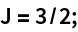In:=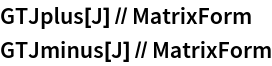Out//MatrixForm=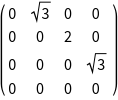Out//MatrixForm=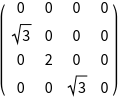Define a state vector
 In:=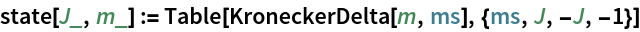Verify action of J+ and normalization.
 In:=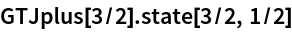Out=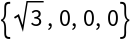In:=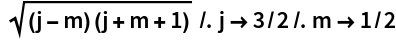Out=Verify action of J+ as a raising operator:
 In:=In:=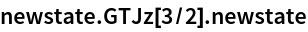Out=GTJTransform applies a symmetry transformation to the basis functions of an irreducible representation of O(3) GTJMatrix gives the representation matrix of a symmetry element for an irreducible representation of O(3)
GTJTransform and GTJMatrix are closely related. While GTJTransform gives the action of a symmetry element on one specific basis function, GTJMatrix gives the transformation matrix of the entire subspace.
 In:=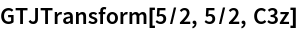Out=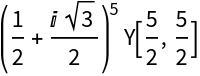In:=Out=In:=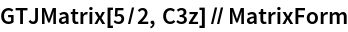Out//MatrixForm=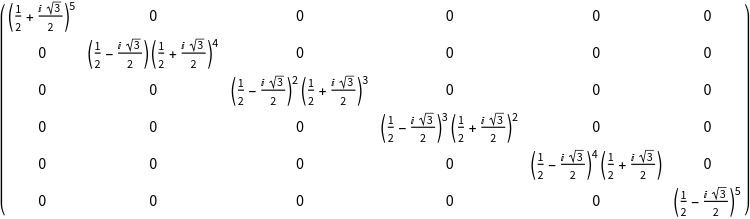GTAngularMomentumRep applies a symmetry transformation to the basis functions of an irreducible representation of O(3) GTAngularMomentumChars gives the representation matrix of a symmetry element for an irreducible representation of O(3)

For the implementation of irreducible representations of O(3), SO(3) and SU(2) we follow .

 Altman, S. L., Rotations, quaternions, and double groups. Chapter 14. Clarendon, 1986

Install the point group Oh (GTInstallGroup)
 In:=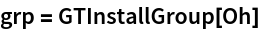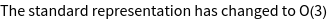Out=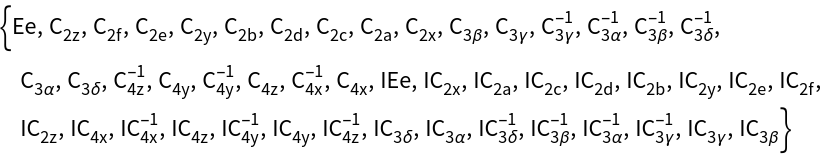Calculate the character Table (GTCharacterTable)
 In:=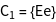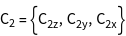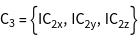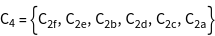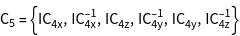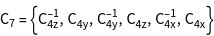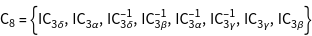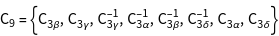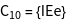Calculate the character system of a single s, p, and d electron
 In:=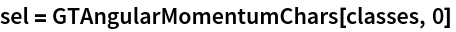Out=In:=Out=In:=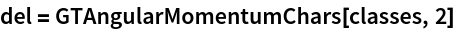Out=Calculate the qualitative splitting in a cubic crystal field
 In:=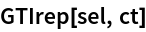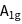Out=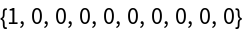In:=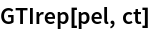Out=In:=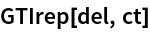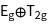Out=Calculate a matrix representation for d-electrons
 In:=In:=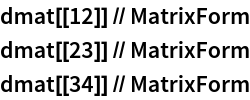Out//MatrixForm=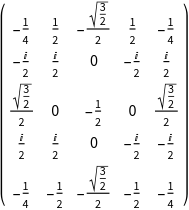Out//MatrixForm=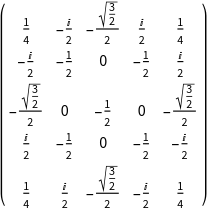Out//MatrixForm=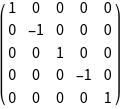Further modifications

GTPack 1.3 contains a new implementation of the modules GTLeftCosets and GTRightCosets. Furthermore, cosets are now ordered by the order of elements. The reordering has also been implemented in GTSGLeftCosets and GTSGRightCosets, respectively. GTGetIreps now outputs names of irreducible representations, e.g., if Mulliken notation is chosen. A new implementation of GTSubGroupQ and the option GOFast has been added.